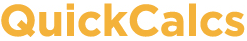1. Select category 2. Choose calculator 3. Enter data 4. View results

## Quantify agreement with kappa

This calculator assesses how well two observers, or two methods, classify subjects into groups. The degree of agreement is quantified by kappa.

### 1. How many categories?

Into how many categories does each observer classify the subjects? For example, choose 3 if each subject is categorized into 'mild', 'moderate' and 'severe'.

Caution: Changing number of categories will erase your data.

### 2. Enter data

Each cell in the table is defined by its row and column. The rows designate how each subject was classified by the first observer (or method). The columns designate how the other observer (or method) classified the subjects. For example, enter into the second row of the first column the number of subjects that the first observer classified into category 'B' and the second observer classified into category 'A'. Enter into the second column of the second row the number of subjects classified by both observers into category 'B'. Subjects categorized identically by both observers are always tallied in the diagonal of the table.

 A B A B